## Helmholtz Differential Equation--Circular Cylindrical Coordinates

In Cylindrical Coordinates, the Scale Factors are,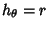,and the separation functions are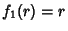,,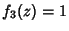, so the Stäckel Determinant is 1. Attempt Separation of Variables by writing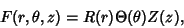(1)

then the Helmholtz Differential Equation becomes(2)

Now divide by,(3)

so the equation has been separated. Since the solution must be periodic infrom the definition of the circular cylindrical coordinate system, the solution to the second part of (3) must have a Negative separation constant(4)

which has a solution(5)

Plugging (5) back into (3) gives(6)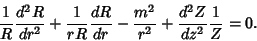(7)

The solution to the second part of (7) must not be sinusoidal at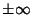for a physical solution, so the differential equation has a Positive separation constant(8)

and the solution is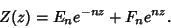(9)

Plugging (9) back into (7) and multiplying through byyields(10)(11)(12)

This is the Bessel Differential Equation, which has a solution(13)

where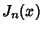and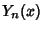are Bessel Functions of the First and Second Kinds, respectively. The general solution is therefore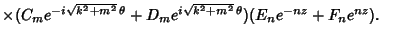(14)

Actually, the Helmholtz Differential Equation is separable for general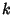of the form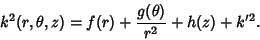(15)